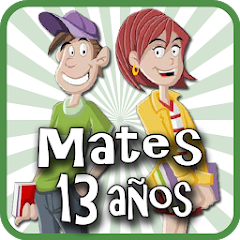# Matemáticas 13 años

10K+Everyone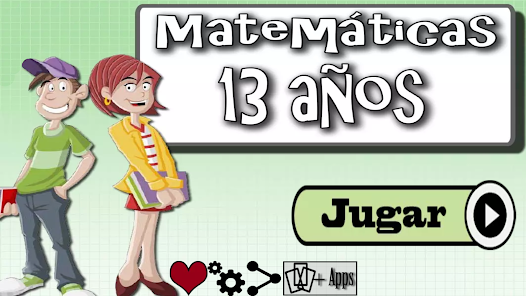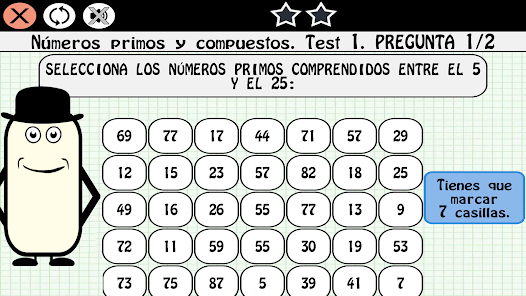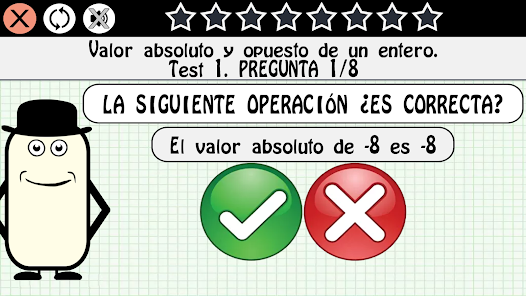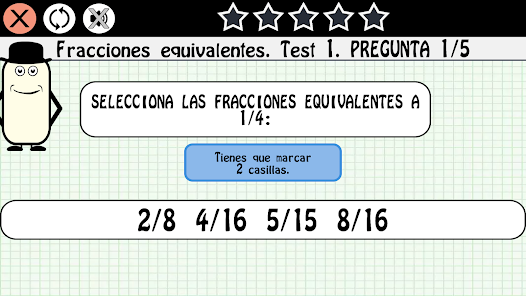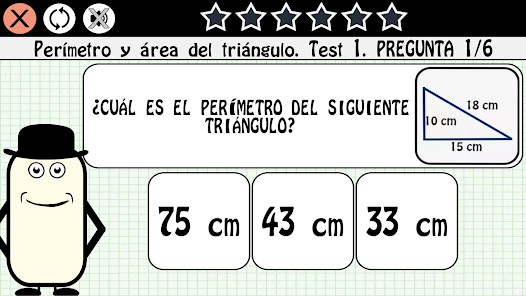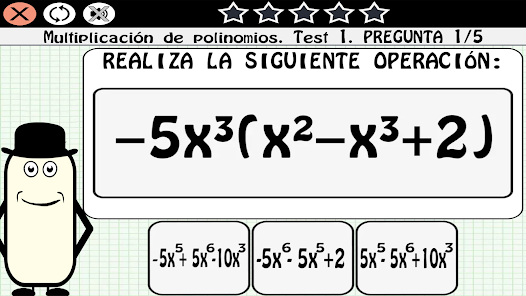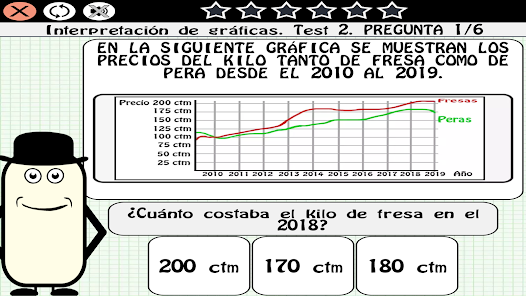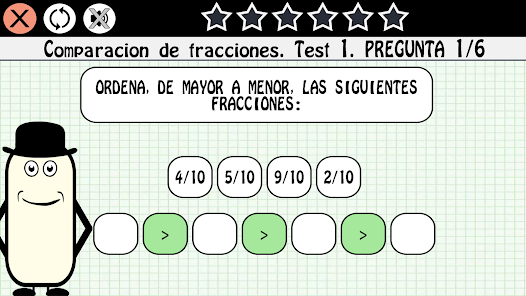Review the MATH syllabus for 13-year-olds with our fun application, completing the subject map until you take the final exam.

- Totally free.
- Specially designed for children in the second year of ESO.
- 1000 questions.
- Final exam.
- Easy to play.
- Possibility to play without Internet.

Syllabus:
Divisibility: Multiples of a number, Least common multiple, Divisors of a number, Greatest common divisor, Prime and composite numbers.
Whole Numbers: Positive and Negative Numbers, Comparison and Ordering of Whole Numbers, Absolute Value and Opposite of an Integer, Addition of Whole Numbers, Whole Number Problems, Multiplication and Division of Whole Numbers, and Powers of Whole Numbers.
Decimal numbers: Decimal numbers, Comparison and ordering of decimals, Approximation by rounding decimals, Addition and subtraction of decimal numbers, Multiplication of decimal numbers and Division of decimal numbers.
Sexagesimal system: Complex and incomplex expressions of measures of time, Problems with quantities of time, Operations with quantities of time, The measure of angles and Operations with measures of angles.
Fractions: Fractions, Fraction of a quantity, Comparison of fractions, Equivalent fractions, Reduction to a common denominator, Addition and subtraction of fractions, Multiplication of fractions and Division of fractions.
Proportionality and percentages: Reduction to the unit, The rule of three, Percentages and Calculation of percentage of a quantity.
Algebra: Addition and subtraction of monomials, Multiplication and division of monomials, Addition and subtraction of polynomials and Multiplication of polynomials.
Equations: Equations and Equation Problems.
Perimeter and area of ​​plane figures: Areas of the square, rectangle, rhombus and rhomboid, Perimeter and area of ​​the triangle, Length of the circumference and Area of ​​the circle.
Geometric bodies: Prisms and pyramids, Polyhedrons and Bodies of revolution.
Graphs of functions: The Cartesian plane and Interpretation of graphs.
Statistics: Bar charts, Mean, Median, Mode, and Range.

Enjoy our application at no cost and reinforce your knowledge of mathematics for 13-year-olds
Updated on
Dec 5, 2022

## Data safety

Safety starts with understanding how developers collect and share your data. Data privacy and security practices may vary based on your use, region, and age. The developer provided this information and may update it over time.No data shared with third parties